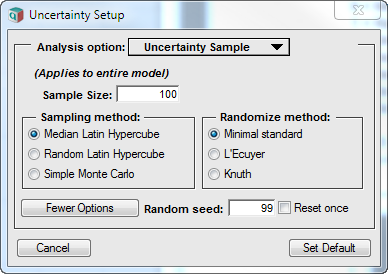# SampleType

(diff) ← Older revision | Latest revision (diff) | Newer revision → (diff)

## SysVar SampleType

Determines the sampling method used to generate samples from probability distributions. Possible values are:

0: Median Latin Hypercube
1: Random Latin Hypercube
2: Simple Monte Carlo
3: biased Median Latin Hypercube (legacy)
4: biased Random latin Hypercube (legacy)
5: Sobol, limiting polynomial order as appropriate for sample size (Analytica 4.6 or higher)
6: Sobol, allow use of high order polynomials (Analytica 4.6 or higher)

## Setting the sample type

In Analytica, you usually set the SampleType from the Uncertainty Settings dialog, by selecting More options on the Uncertainty Sample tab.The dialog gives you access to options 0, 1 and 2 only. To select options 3 thru 6, you need to set the system variable from the Typescript Window.

## Latin Hypercube

Latin hypercube methods generate a sample of size N for a scalar uncertainty by selecting one sample from each [(i - 1)/N, i/N]-fractile interval, i = 1..N. For example, with a sample size of 5, Latin Hypercube would ensure that one point is in the 0 to 20th percentile range, another point between the 20th to 40th percentiles, one between the 40th to 60th percentiles, one between the 60th to 80th percentiles and in the 80th to 100th percentile range. This helps to ensure that the full range of values gets coverage an avoid random clumping that can occur with pure Monte Carlo. The points within the sample are randomly shuffled for each scalar uncertainty.

Median Latin Hypercube (MLH) selects the median percentile from each percentile range. This ensures maximal spreading of the sample points, but it also means that the set of samples is deterministic -- you'll always get the same points in the sample. The order of the points along the Run index is random, since the points are shuffled, so the sample itself (taking ordering into account) does indeed have a random component.

Random Latin Hypercube (RLH) selects the point in each percentile range at random.

Latin Hypercube methods ensure spreading of the sample points within each individual scalar dimension, but there is no coordination between separate scalar uncertainties. Hence, in a 2-D sample space, clumping and areas with minimal coverage are still possible. Latin Hypercube methods converge quadratically faster than pure Month Carlo for smooth (analytic) problems involving a single uncertainty. No theoretical guarantees exist when multiple scalar uncertainties are involved, but measurably better convergence is often observed in real-life models with as many as 40 scalar uncertainties (see Latin Hypercube vs. Monte Carlo sampling). With a large number of scalar uncertainties, convergence rates are not substantially better than Monte Carlo.

In non-smooth models, Latin Hypercube methods can on rare occasions produce artifacts that slow convergence to be worse that Monte Carlo. These situations are rare.

## Monte Carlo

Pure Monte Carlo samples every point independently. It is a classical method in statistics, for which much is known. It lends itself to proof of many theoretical properties. Sampling error decreases as $\displaystyle{ 1/\sqrt{N} }$, so that to halve the sampling error, you need to quadruple the sample size.

## Biased Latin Hypercube

Options 3 and 4 exist only to reproduce the same samples returned in Analytica 4.2 and earlier, but should not be used otherwise.

In these earlier versions of Analytica, there was a very small bias in the shuffling algorithm.

## Sobol sequences

Sobol sequences are a quasi-Monte Carlo method that can converge much faster than simple Monte Carlo or Latin hypercube for many models. Sobol sequences attempt to distribute points evenly in the multi-dimensional sample space of multiple scalar uncertainties. Latin Hypercube methods distribute the samples more evenly than Monte Carlo over each uncertain variable (or dimension) viewed separately, but their advantage reduces when applied to models with multiple uncertain variables. Sobol sequences spread the distribution more evenly over the multidimensional sample space, and so can perform better with multiple uncertainties.

Sobol sequences come with a theoretical guarantee that the sampling error converges as:

$\displaystyle{ O((\log N)^d / N ) }$

where d is the number of scalar uncertainties and «N» is the sample size. As «N» gets large, the denominator dominates the numerator, bringing this close to the holy grail of $\displaystyle{ O(1/N) }$ -- which would be quadratically faster than Monte Carlo. However, when «d» is large, as it often is, the numerator in this formula is huge, and indeed the improved performance is often not evident until sample sizes approach astronomical sizes.

Many publications claim to have created algorithms superior to Monte Carlo and Latin hypercube by using Sobol sampling. Lonnie Chrisman's experiments find that it is not substantially better than Latin Hypercube, and sometimes notably worse for realistic sample sizes (i.e., sample sizes under 1 million).

There are two Sobol methods provided. Option 5 is the better of the two. It limits the highest order Sobol polynomial based on sample size. It also limits the number of scalar uncertainties that are "coordinated" before it starts over again with low-order polynomials. Option 6 does not limit the polynomial order, allowing up to 21,000 scalar Sobol dimensions simultaneously before starting over again with the low-order polynomials. With option 6 many artifacts occur in which points do not get even spread through the high-dimensional space, and obvious sections of the space go unexplored. These artifacts would disappear with enough sample points -- method 5 excludes the high-order Sobol polynomials that don't have a sample size large enough to support them, making it work much better.